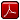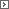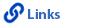Mathematics Grade 7Printable Version (pdf)Course Introduction

Core Standards of the Course

Strand: MATHEMATICAL PRACTICES (7.MP)
The Standards for Mathematical Practice in Seventh Grade describe mathematical habits of mind that teachers should seek to develop in their students. Students become mathematically proficient in engaging with mathematical content and concepts as they learn, experience, and apply these skills and attitudes (Standards 7.MP.1�8).Standard 7.MP.1
Make sense of problems and persevere in solving them. Explain the meaning of a problem and look for entry points to its solution. Analyze givens, constraints, relationships, and goals. Make conjectures about the form and meaning of the solution, plan a solution pathway, and continually monitor progress asking, "Does this make sense?" Consider analogous problems, make connections between multiple representations, identify the correspondence between different approaches, look for trends, and transform algebraic expressions to highlight meaningful mathematics. Check answers to problems using a different method.

Standard 7.MP.2
Reason abstractly and quantitatively. Make sense of the quantities and their relationships in problem situations. Translate between context and algebraic representations by contextualizing and decontextualizing quantitative relationships. This includes the ability to decontextualize a given situation, representing it algebraically and manipulating symbols fluently as well as the ability to contextualize algebraic representations to make sense of the problem.

Standard 7.MP.3
Construct viable arguments and critique the reasoning of others. Understand and use stated assumptions, definitions, and previously established results in constructing arguments. Make conjectures and build a logical progression of statements to explore the truth of their conjectures. Justify conclusions and communicate them to others. Respond to the arguments of others by listening, asking clarifying questions, and critiquing the reasoning of others.

Standard 7.MP.4
Model with mathematics. Apply mathematics to solve problems arising in everyday life, society, and the workplace. Make assumptions and approximations, identifying important quantities to construct a mathematical model. Routinely interpret mathematical results in the context of the situation and reflect on whether the results make sense, possibly improving the model if it has not served its purpose.

Standard 7.MP.5
Use appropriate tools strategically. Consider the available tools and be sufficiently familiar with them to make sound decisions about when each tool might be helpful, recognizing both the insight to be gained as well as the limitations. Identify relevant external mathematical resources and use them to pose or solve problems. Use tools to explore and deepen their understanding of concepts.

Standard 7.MP.6
Attend to precision. Communicate precisely to others. Use explicit definitions in discussion with others and in their own reasoning. They state the meaning of the symbols they choose. Specify units of measure and label axes to clarify the correspondence with quantities in a problem. Calculate accurately and efficiently, express numerical answers with a degree of precision appropriate for the problem context.

Standard 7.MP.7
Look for and make use of structure. Look closely at mathematical relationships to identify the underlying structure by recognizing a simple structure within a more complicated structure. See complicated things, such as some algebraic expressions, as single objects or as being composed of several objects. For example, see 5 � 3(x � y)2 as 5 minus a positive number times a square and use that to realize that its value cannot be more than 5 for any real numbers x and y.

Standard 7.MP.8
Look for and express regularity in repeated reasoning. Notice if reasoning is repeated, and look for both generalizations and shortcuts. Evaluate the reasonableness of intermediate results by maintaining oversight of the process while attending to the details.

Strand: RATIOS AND PROPORTIONAL RELATIONSHIPS (7.RP)
Analyze proportional relationships and use them to solve real-world and mathematical problems (Standards 7.RP.1�3).Standard 7.RP.1
Compute unit rates associated with ratios of fractions, including ratios of lengths, areas and other quantities measured in like or different units. For example, if a person walks 1/2 mile in each 1/4 hour, compute the unit rate as the complex fraction 1/2/1/4 miles per hour, equivalently 2 miles per hour.Standard 7.RP.2
Recognize and represent proportional relationships between quantities.1. Decide whether two quantities are in a proportional relationship, e.g., by testing for equivalent ratios in a table or graphing on a coordinate plane and observing whether the graph is a straight line through the origin.
2. Identify the constant of proportionality (unit rate) in tables, graphs, equations, diagrams, and verbal descriptions of proportional relationships.
3. Represent proportional relationships by equations. For example, if total cost t is proportional to the number n of items purchased at a constant price p, the relationship between the total cost and the number of items can be expressed as t = pn.
4. Explain what a point (x, y) on the graph of a proportional relationship means in terms of the situation, with special attention to the points (0, 0) and (1, r) where r is the unit rate.

Standard 7.RP.3
Use proportional relationships to solve multistep ratio and percent problems. Examples: simple interest, tax, markups and markdowns, gratuities and commissions, fees, percent increase and decrease, percent error.Strand: THE NUMBER SYSTEM (7.NS)
Apply and extend previous understandings of operations with fractions to add, subtract, multiply, and divide rational numbers (Standards 7.NS.1�3).Standard 7.NS.1
Apply and extend previous understandings of addition and subtraction to add and subtract rational numbers; represent addition and subtraction on a horizontal or vertical number line diagram.1. Describe situations in which opposite quantities combine to make 0. For example, a hydrogen atom has 0 charge because its two constituents are oppositely charged.
2. Understand p + q as the number located a distance |q | from p, in the positive or negative direction depending on whether q is positive or negative. Show that a number and its opposite have a sum of 0 (are additive inverses). Interpret sums of rational numbers by describing real-world contexts.
3. Understand subtraction of rational numbers as adding the additive inverse, pq = p + (–q). Show that the distance between two rational numbers on the number line is the absolute value of their difference, and apply this principle in real-world contexts.
4. Apply properties of operations as strategies to add and subtract rational numbers.

Standard 7.NS.2
Apply and extend previous understandings of multiplication and division and of fractions to multiply and divide rational numbers.1. Understand that multiplication is extended from fractions to rational numbers by requiring that operations continue to satisfy the properties of operations, particularly the distributive property, leading to products such as (–1)(–1) = 1 and the rules for multiplying signed numbers. Interpret products of rational numbers by describing real-world contexts.
2. Understand that integers can be divided, provided that the divisor is not zero, and every quotient of integers (with non-zero divisor) is a rational number. If p and q are integers, then –(p/q) = (–p)/q = p/(–q). Interpret quotients of rational numbers by describing real-world contexts.
3. Apply properties of operations as strategies to multiply and divide rational numbers.
4. Convert a rational number to a decimal using long division; know that the decimal form of a rational number terminates in 0s or eventually repeats.

Standard 7.NS.3
Solve real-world and mathematical problems involving the four operations with rational numbers. Computations with rational numbers extend the rules for manipulating fractions to complex fractions.Strand: EXPRESSIONS AND EQUATIONS (7.EE)
Use properties of operations to generate equivalent expressions (Standards 7.EE.1�2). Solve real-life and mathematical problems using numerical and algebraic expressions and equations (Standards 7.EE.3�4).Standard 7.EE.1
Apply properties of operations as strategies to add, subtract, factor, and expand linear expressions with rational coefficients.Standard 7.EE.2
Understand that rewriting an expression in different forms in a problem context can shed light on the problem and how the quantities in it are related. For example, a + 0.05a = 1.05a means that “increase by 5%” is the same as “multiply by 1.05.”Standard 7.EE.3
Solve multi-step real-life and mathematical problems posed with positive and negative rational numbers in any form (whole numbers, fractions, and decimals), using tools strategically. Apply properties of operations to calculate with numbers in any form; convert between forms as appropriate; and assess the reasonableness of answers using mental computation and estimation strategies. For example: If a woman making \$25 an hour gets a 10% raise, she will make an additional 1/10 of her salary an hour, or \$2.50, for a new salary of \$27.50. If you want to place a towel bar 9 3/4 inches long in the center of a door that is 27 1/2 inches wide, you will need to place the bar about 9 inches from each edge; this estimate can be used as a check on the exact computation.Standard 7.EE.4
Use variables to represent quantities in a real-world or mathematical problem, and construct simple equations and inequalities to solve problems by reasoning about the quantities.1. Solve word problems leading to equations of the form px + q = r and p(x + q) = r, where p, q, and r are specific rational numbers. Solve equations of these forms fluently. Compare an algebraic solution to an arithmetic solution, identifying the sequence of the operations used in each approach. For example, the perimeter of a rectangle is 54 cm. Its length is 6 cm. What is its width?
2. Solve word problems leading to inequalities of the form px + q > r or px + q < r, where p, q, and r are specific rational numbers. Graph the solution set of the inequality and interpret it in the context of the problem. For example: As a salesperson, you are paid \$50 per week plus \$3 per sale. This week you want your pay to be at least \$100. Write an inequality for the number of sales you need to make, and describe the solutions.

Strand: GEOMETRY (7.G)
Draw, construct, and describe geometrical figures, and describe the relationships between them (Standards 7.G.1�3). Solve real-life and mathematical problems involving angle measure, area, surface area, and volume (Standards 7.G.4�6).Standard 7.G.1
Solve problems involving scale drawings of geometric figures, including computing actual lengths and areas from a scale drawing and reproducing a scale drawing at a different scale.Standard 7.G.2
Draw (freehand, with ruler and protractor, and with technology) geometric shapes with given conditions. Focus on constructing triangles from three measures of angles or sides, noticing when the conditions determine a unique triangle, more than one triangle, or no triangle.Standard 7.G.3
Describe the two-dimensional figures that result from slicing three-dimensional figures, as in plane sections of right rectangular prisms and right rectangular pyramids.Standard 7.G.4
Know the formulas for the area and circumference of a circle and use them to solve problems; give an informal derivation of the relationship between the circumference and area of a circle.Standard 7.G.5
Use facts about supplementary, complementary, vertical, and adjacent angles in a multi-step problem to write and solve simple equations for an unknown angle in a figure.Standard 7.G.6
Solve real-world and mathematical problems involving area, volume and surface area of two- and three-dimensional objects composed of triangles, quadrilaterals, polygons, cubes, and right prisms.Strand: STATISTICS AND PROBABILITY (7.SP)
Use random sampling to draw inferences about a population (Standards 7.SP.1�2). Draw informal comparative inferences about two populations (Standards 7.SP.3�4). Investigate chance processes and develop, use, and evaluate probability models (Standards 7.SP.5�8).Standard 7.SP.1
Understand that statistics can be used to gain information about a population by examining a sample of the population; generalizations about a population from a sample are valid only if the sample is representative of that population. Understand that random sampling is more likely to produce representative samples and support valid inferences.Standard 7.SP.2
Use data from a random sample to draw inferences about a population with an unknown characteristic of interest. Generate multiple samples (or simulated samples) of the same size to gauge the variation in estimates or predictions. For example, estimate the mean word length in a book by randomly sampling words from the book; predict the winner of a school election based on randomly sampled survey data. Gauge how far off the estimate or prediction might be.Standard 7.SP.3
Informally assess the degree of visual overlap of two numerical data distributions with similar variabilities, measuring the difference between the centers by expressing it as a multiple of a measure of variability. For example, the mean height of players on the basketball team is 10 cm greater than the mean height of players on the soccer team, approximately twice the variability (mean absolute deviation) on either team; on a dot plot, the separation between the two distributions of heights is noticeable.Standard 7.SP.4
Use measures of center and measures of variability for numerical data from random samples to draw informal comparative inferences about two populations. For example, decide whether the words in a chapter of a seventh-grade science book are generally longer than the words in a chapter of a fourth-grade science book.Standard 7.SP.5
Understand that the probability of a chance event is a number between 0 and 1 that expresses the likelihood of the event occurring. Larger numbers indicate greater likelihood. A probability near 0 indicates an unlikely event, a probability around 1/2 indicates an event that is neither unlikely nor likely, and a probability near 1 indicates a likely event.Standard 7.SP.6
Approximate the probability of a chance event by collecting data on the chance process that produces it and observing its long-run relative frequency, and predict the approximate relative frequency given the probability. For example, when rolling a number cube 600 times, predict that a 3 or 6 would be rolled roughly 200 times, but probably not exactly 200 times.Standard 7.SP.7
Develop a probability model and use it to find probabilities of events. Compare probabilities from a model to observed frequencies; if the agreement is not good, explain possible sources of the discrepancy.1. Develop a uniform probability model by assigning equal probability to all outcomes, and use the model to determine probabilities of events. For example, if a student is selected at random from a class, find the probability that Jane will be selected and the probability that a girl will be selected.
2. Develop a probability model (which may not be uniform) by observing frequencies in data generated from a chance process. For example, find the approximate probability that a spinning penny will land heads up or that a tossed paper cup will land open-end down. Do the outcomes for the spinning penny appear to be equally likely based on the observed frequencies?

Standard 7.SP.8
Find probabilities of compound events using organized lists, tables, tree diagrams, and simulation.1. Understand that, just as with simple events, the probability of a compound event is the fraction of outcomes in the sample space for which the compound event occurs.
2. Represent sample spaces for compound events using methods such as organized lists, tables and tree diagrams. For an event described in everyday language (e.g., “rolling double sixes”), identify the outcomes in the sample space which compose the event.
3. Design and use a simulation to generate frequencies for compound events. For example, use random digits as a simulation tool to approximate the answer to the question: If 40% of donors have type A blood, what is the probability that it will take at least 4 donors to find one with type A blood?http://www.uen.org - in partnership with Utah State Board of Education (USBE) and Utah System of Higher Education (USHE).  Send questions or comments to USBE Specialist - Lindsey  Henderson and see the Mathematics - Secondary website. For general questions about Utah's Core Standards contact the Director - Jennifer  Throndsen.

These materials have been produced by and for the teachers of the State of Utah. Copies of these materials may be freely reproduced for teacher and classroom use. When distributing these materials, credit should be given to Utah State Board of Education. These materials may not be published, in whole or part, or in any other format, without the written permission of the Utah State Board of Education, 250 East 500 South, PO Box 144200, Salt Lake City, Utah 84114-4200.